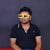# Sorting — Algorithm- javascript

1. Bubble Sorting Algorithm:
`function bubbleSort2(a) {  for (let i = 0; i < a.length; i++) {    for (let j = 0; j < a.length; j++) {      if (a[j] > a[j + 1]) {        temp = a[j];        a[j] = a[j + 1];        a[j + 1] = temp;      }    }  }  return a;}`

# Write your pollyfill of Map, Call , Apply, Bind without help of es6

`Array.prototype.myMap = function (callback) { const newArray = []; for(const item of this) { newArray.push(callback(item)); } return newArray;};`
`Function.prototype.myCall = function(obj) {   obj = obj || global;   var id = "00" + Math.random();  while (obj.hasOwnProperty(id)) {    id = "00" + Math.random();  }  obj[id] = this;  let arg=[];  for(let i=1;i<arguments.length;i++){       arg.push("arguments[" + i + "]");  } result= eval("obj[id]("+arg+")");  delete obj[id];  return result;}`
`Function.prototype.myApply = function(obj, argArr) { obj = obj || global; var id = "00" + Math.random(); while (obj.hasOwnProperty(id)) {  id = "00" + Math.random(); } obj[id] = this; let arg=[]…`

# Test Case using Enzyme: React Component

## Assuming you have reactjs application configured,we are going to define component in react js and will test it using enzyme.